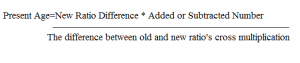# HOW TO SOLVE PROBLEMS ON AGES -PART 2

Hi Bankersdaily Aspirant,

Aspirants today we are going to discuss on Problems based on Ages,As you all know IBPS RRB exams are going to held shortly and we are discussing about each topic regularly with their shortcuts.Click to learn each topic with their shortcuts

Today,we are going to discuss on how to solve Problems based on Ages Part-2

### INTRODUCTION:

Problems based on Ages is the easiest topic in all the banking exams,and it is asked in Prelims and Mains Exam for 1 or 2 Mark.

There are only 3 Types of problems in ages namely

1.Finding  Present Age

2.Finding  Previous Age(Age before some years)

3.Finding  Future Age(Age after Some years)

We had discussed how to solve problems based on ages in the previous article,Click here to view how to solve problems based on Ages

Note :

If they didn’t specify the age then consider the age to be X

### FINDING AGE BEFORE SOME YEARS :

In this type we are going to assume the present age is X and to find the age before   Y  years then  Age=X-Y

1)Ganesh is 24 years younger than his Father Bholenath.3 years ago,Ganesh was 1/5 as old as his father was.What is Ganesh’s present age?

Explanation:
 Years Bholenath Ganesh Present X X-24 3 years Ago X-3 X-24-3=X-27

Before 3 years:

X-27=1/5(X-3)

5X-135=X-3

4X=132

X=33

Ganesh’s Age =(33-24)=9 Years

2.The ratio of ages of Amir and Salman is at present 3:4, 5 Years before that ratio was 2:3.What is the present age of Amir and Salman?

Explanation:
 Years Amir Salman Present Year 3X 4X 5 years ago 2 3

(3X-5)/(4X-5)=2/3   ⇒    X=5

Amir’s Present Age=15years  and Salman’s Present Age=20years

### FINDING AGES AFTER SOME YEARS:

Let the number of years be X and the age after Y  years is X+Y years

1.A person’s Present Age is two-fifth of the age of his mother. After 8 years, he will be one-half of the age of his mother. How old is the mother at present?

Explanation:

Let the present age of the mother be X years,

Then, the present age of the son is 2/5(X) years.

[2/5(X)+8]=1/2[X+8]

2(2X+40)=5(X+8)

X=40

2.Ranjan’s  and  Anurag’s Age are in the ratio  4:5.4Years hence,their age ratio will become 5:6.What is Anurag’s present age?

Explanation:
 YEARS ANURAG RANJAN Present year 5 4 After 4 year 6 5

=

X=4

Anurag’s Present Age=20 years

a)16  b)20  c)25  d)30

Alternate Method(Choosing  from the option)

Ratio’s are 4:5  and  after 4 years 5:6

It is clear that Anurag age is a multiple of 4 now And after 4 yrs it is a multiple of 5

### SHORTCUT  METHOD:

When two ratio’s and after/before some years are given

Formula1.The age of Rahul and Amit are in the ratio of  8:7.After 10 years the ratio of their Ages will be 13:12.What is the difference in years between their ages?

Explanation:

New Ratio Difference=13-12=1

Difference between old and new ratio’s Cross Multiplication

=(8/7) * (13/12)

= (96-91)=5

Present Age=(1*10)/5  = 2yrs

Difference between their age =(8*2)-(7*2)=16years-14years=2years